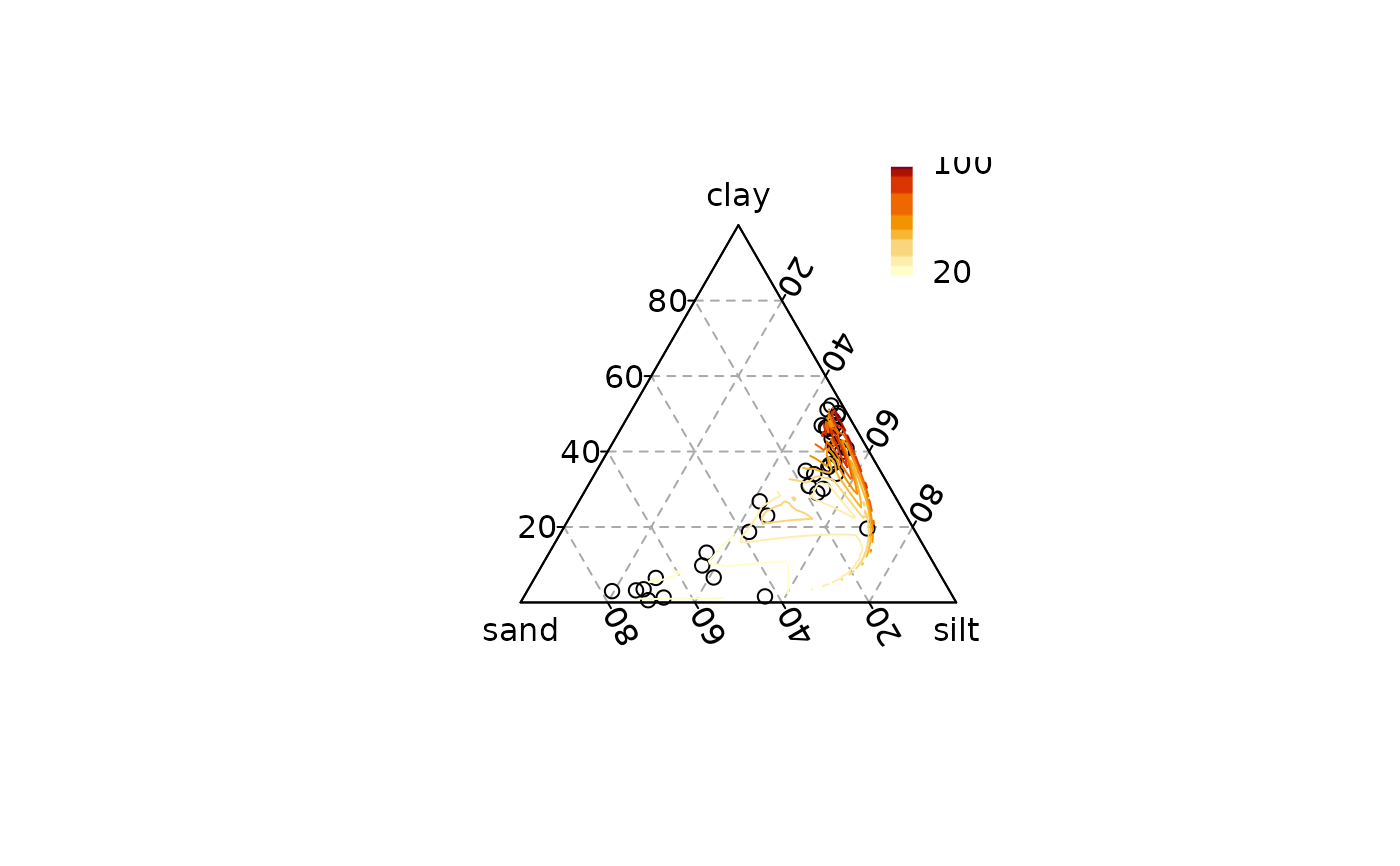Computes and draws contour lines.

## Usage

ternary_contour(x, y, z, ...)

# S4 method for numeric,numeric,numeric
ternary_contour(
x,
y,
z,
value,
n = 50,
nlevels = 10,
levels = pretty(range(value, na.rm = TRUE), nlevels),
ilr = TRUE,
linear = TRUE,
extrapolate = FALSE,
palette = function(i) grDevices::hcl.colors(i, "YlOrRd", rev = TRUE),
...
)

# S4 method for ANY,missing,missing
ternary_contour(
x,
value,
n = 50,
nlevels = 10,
levels = pretty(range(value, na.rm = TRUE), nlevels),
ilr = TRUE,
linear = TRUE,
extrapolate = FALSE,
palette = function(i) grDevices::hcl.colors(i, "YlOrRd", rev = TRUE),
...
)

## Arguments

x, y, z

A numeric vector giving the x, y and z ternary coordinates of a set of points. If y and z are missing, an attempt is made to interpret x in a suitable way (see grDevices::xyz.coords()).

...

Further arguments to be passed to ternary_lines().

value

A numeric vector giving the values to be plotted.

n

A length-one numeric specifying the number of grid points.

nlevels

A length-one numeric vector specifying the number of contour levels desired. Only used if levels is NULL.

levels

A numeric vector of levels at which to draw contour lines.

ilr

A logical scalar: should interpolation be computed in ILR space? If FALSE, interpolation is computed in Cartesian space.

linear

A logical scalar: should linear interpolation be used? If FALSE, spline interpolation is used (see akima::interp()).

extrapolate

A logical scalar: should extrapolation be used outside of the convex hull determined by the data points (see akima::interp())?

palette

A color palette function that takes a single integer argument (the number of levels) and returns a vector of colors.

## Value

ternary_contour() is called it for its side-effects.

Invisibly returns a list with elements levels (the contour levels) and colors (the contour colors) that can be used for a legend.

## Details

Contour are computed from a bivariate interpolation onto a grid, after an isometric log ratio transformation of the original data.

## Note

The akima package needs to be installed on your machine.

akima::interp(), grDevices::contourLines()

Other statistics: ternary_density(), ternary_ellipse(), ternary_hull(), ternary_mean(), ternary_pca()

N. Frerebeau

## Examples

## Add density
## Data from Aitchison 1986
ternary_plot(arctic, panel.first = ternary_grid())
levels <- ternary_contour(arctic, value = arctic$depth, n = 100, nlevels = 10) ## Add a legend legend_image <- grDevices::as.raster(rev(levels$colors))
graphics::rasterImage(legend_image, 0.85, 0.75, 0.9, 1)
graphics::text(x = 0.9, y = c(0.75, 1), labels = range(levels\$levels), pos = 4)